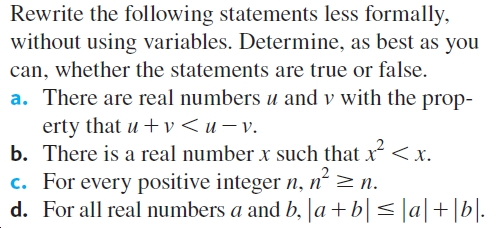# Rewrite the following statements less formally,without using variables. Determine, as best as youcan, whether the statements are true or false.a. There are real numbers u and v with the prop-erty that u + v < u v.b. There is a real number x such that x< x.c. For every positive integer n, n² >n.d. For all real numbers a and b, |a+b|< ]a|+[b|.

Question
4 viewshelp_outlineImage TranscriptioncloseRewrite the following statements less formally, without using variables. Determine, as best as you can, whether the statements are true or false. a. There are real numbers u and v with the prop- erty that u + v < u v. b. There is a real number x such that x< x. c. For every positive integer n, n² >n. d. For all real numbers a and b, |a+b|< ]a|+[b|. fullscreen
check_circle

Step 1

(a) The given statement is true.

For example, take

Step 2

Hence, there exists real numbers u and v such that their sum is less than their difference.

Less formally, we can write there exists two real numbers such that their sum is less than the difference between them.

(b) The given statement is true.

Step 3

Specifically, this is true for any x lying between -1 and 1.

Less formally, we can write there exists a real number whose square is l...

### Want to see the full answer?

See Solution

#### Want to see this answer and more?

Solutions are written by subject experts who are available 24/7. Questions are typically answered within 1 hour.*

See Solution
*Response times may vary by subject and question.
Tagged in

### Math